Previous: 2.1.1 The Boltzmann Equation Up: 2.1 The Boltzmann Poisson Next: 2.1.3 Dimensional Reduction

## 2.1.2 Poisson Equation

By definition the potential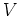fulfills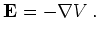(2.7)

By Maxwell's equations we have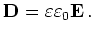(2.8)

and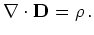(2.9)

Here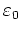is the electrical permittivity of free space and the dimensionless dielectric constant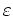is a material dependent parameter. In our case the charge density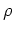is given by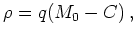(2.10)

with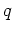the (negative) elementary charge,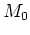the zeroth moment, that is, the particle density, and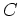the net doping.